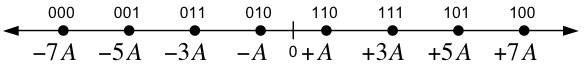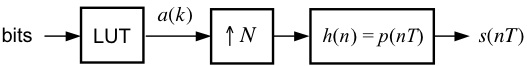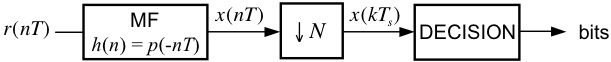EECS 700: M-ary Pulse Amplitude Modulation (MPAM)

Objective
The goal of this exercise is to correctly demodulate the noisy received signal in Rx8PAM.mat.  The major new "step" in this exercise is the need for proper DECISION logic (not just a simple SIGN operation).

Signal Constellation

The transmitted data points are taken from the signal space constellation below.  The 8 points are equally spaced on a line with a spacing of 2A.
Each transmitted signal carries three information bits, as shown below.After being corrupted by noise, the received signal is demodulated by the system below, which detects the transmitted signal space points and outputs the estimated values of the transmitted bits.

Design Exercise

Part I: Generic MPAM TransmitterUsing blocks from the SIMULINK Block Library, the Signal Processing Blockset, and the Communications Blockset, design a MPAM modulator, patterned after the one shown above, to meet the following specifications:
Number of samples per symbol: 16
Pulse shape:                           HS
Average energy:                      84

As you can see, the above modulator is identical to the Binary PAM Modulator.  The only difference between modulators for M=2 and M-arbitrary is the contents of the respective look-up tables (in fact, the binary PAM case was so simple, we did not need a look-up table).

Part II: Generic MPAM DetectorUsing blocks from the SIMULINK Block Library, the Signal Processing Blockset, and the Communications Blockset, design a MPAM detector, patterned after the one shown above, that is compatible with the modulator from Part I.  In your implementation, you might find it eaiser to output the signal space projections, x(kTs), to the MATLAB workspace and implement the decision logic in a post-processing fashion using a MATLAB script or MATLAB function.  Adding the functionality of the DECISION block is the only thing that is really "new" in this lab exercise.

[ Sanity Check ]
You can test your designs from Parts I and II by connecting the output of your modulator to the input of your detector.  The data source (Signal from Workspace block) emits symbol indexes, which are integers in the range 0 to 7 (each of these integers has a 3-bit representation).  Set this block to output the four-symbol sequence [0 2 5 7]'.  The look-up table converts these indexes to the constellation points.  In your project window, go to the menu Simulation --> Configuration Parameters and set the parameters to:
Start Time:         0.0
Stop Time:          (4+1)*16
Solver options:    Type: Fixed-step, Solver: discrete (no continuous states)
Fixed step size:    1
Note: The 4 corresponds to the number of transmitted symbols, the 1 corresponds to the delay of the downsample operation in the detector, and the 16 is the number of samples per symbol.

Remember, it is very important that your Downsample block is sampling at the correct "phase".  This may require some adjustment/experimentation on your part.  If you plot an eye diagram, this will tell you if your implementation is correct enough, because you will be able to see the correct sampling instant.   After that, it is just a matter of adjusting the sampling phase so that you sample these points. You want to sample the matched filter outputs when the signal passes exactly through the constellation points. When you have successfully debugged your system, you are ready for the final part of the exercise.

Part III: Detecting an Unknown Data Set

Here are the steps for the final part of the exercise:
1. Connect the input of your detector to a From File block and set the filename to Rx8PAM.mat (If you have problems with that file, here is a ZIP version).
2. Set the simulation parameters to:
Start Time:         0.0
Stop Time:          (42+1)*16
Solver options:    Type: Fixed-step, Solver: discrete (no continuous states)
Fixed step size:    1
3. Run the Simulation.
4. The last 42 values of the sampled matched filter outputs represent 18 ASCII characters.  Determine the message using your own conversion script or an ASCII table, such as the one found here.
5. Submit your answer AND your detector model file (.mdl SIMULINK file) to the class TA via the Digital Drop Box in Blackboard (you may submit via e-mail only if you have problems with Blackboard).  You should organize your files into a folder, and then ZIP the folder and submit the ZIP file.  The naming convention for the ZIP file is "Lab#_YourLastName.zip."
6. Plot the eye diagram and signal space projections.  You may submit these electronically, or turn them in at the beginning of the next class period after the due date.

Back to the Lab Exercises Page